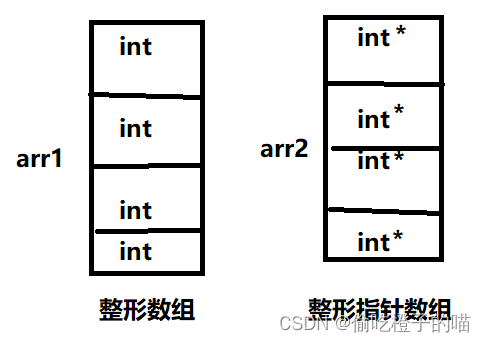• 指针运算（补）
• 指针+指针
• 指针的关系运算（补）
• 指针与数组
• 数组名
• 二级指针
• 指针数组

# 指针运算（补）

## 指针+指针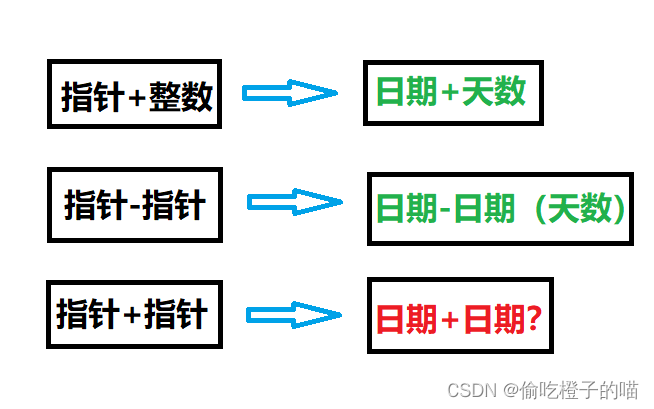## 指针的关系运算（补）

``````#define N_VALUES 5
int main()
{
int values[N_VALUES] = { 0,1,2,3,4 };
int* vp = 0;
for (vp = &values[N_VALUES]; vp > &values;)
{
*--vp = 0;
printf("%d", *vp);
}
}
``````

``````#define N_VALUES 5
int main()
{
int values[N_VALUES] = { 0,1,2,3,4 };
int* vp = 0;
for (vp = &values[N_VALUES]; vp > &values;vp--)
{
*vp = 0;
printf("%d", *vp);
}
}
``````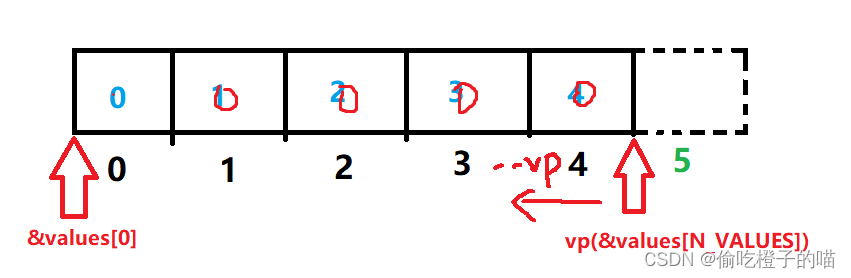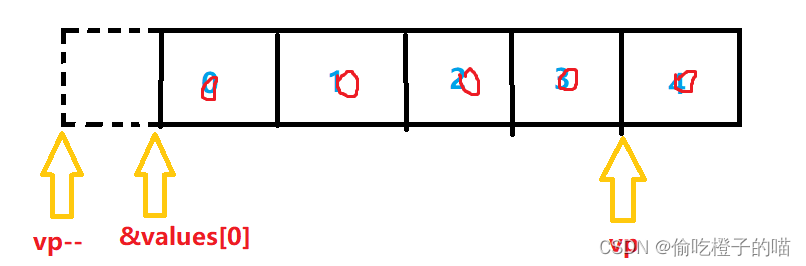# 指针与数组

## 数组名

``````int main()
{
int arr = { 0 };
printf("%p\n", arr);
printf("%p\n", &arr);

return 0;
}
``````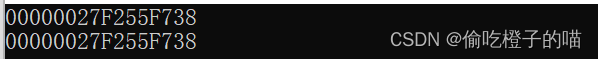``````int main()
{
int arr[] = {1,2,3,4,5,6,7,8,9,0};
int *p = arr; //指针存放数组首元素的地址
int sz = sizeof(arr)/sizeof(arr);
for(i=0; i<sz; i++)
{
printf("&arr[%d] = %p   <====> p+%d = %p\n", i, &arr[i], i, p+i);
}
return 0;
}
``````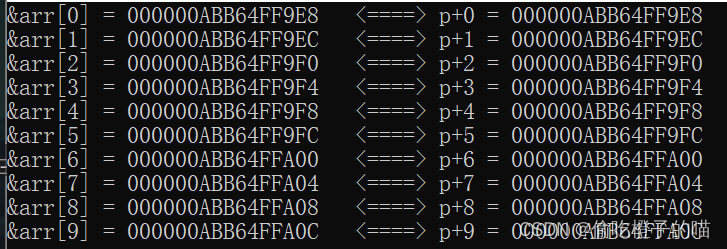``````int main()
{
int arr[] = { 1, 2, 3, 4, 5, 6, 7, 8, 9, 0 };
int *p = arr; //指针存放数组首元素的地址
int sz = sizeof(arr) / sizeof(arr);
int i = 0;
for (i = 0; i<sz; i++)
{
printf("%d ", *(p + i));
}
return 0;
}
``````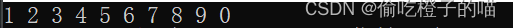## 二级指针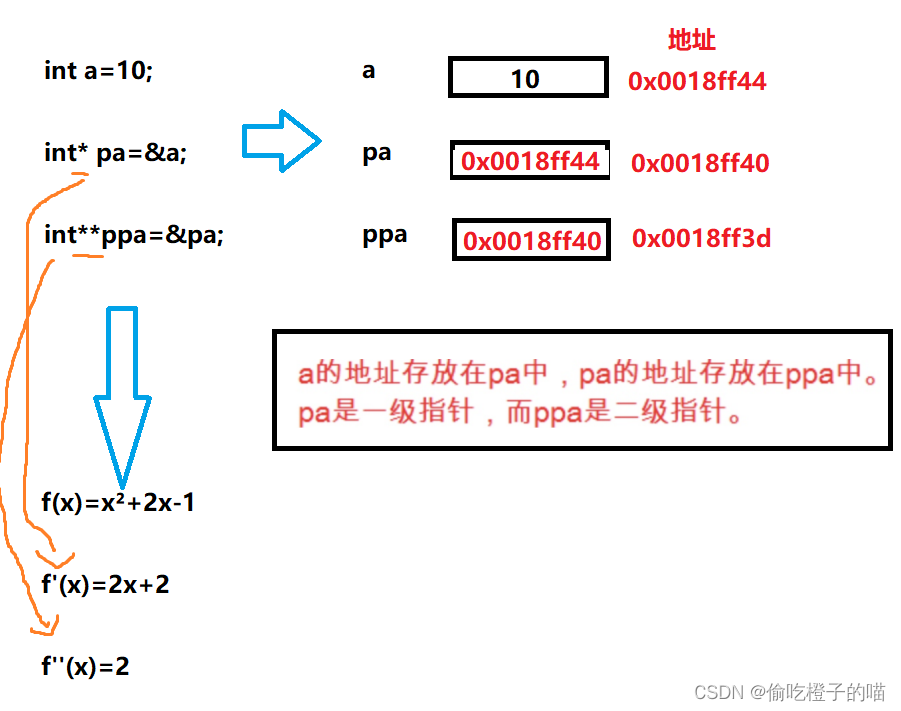## 指针数组